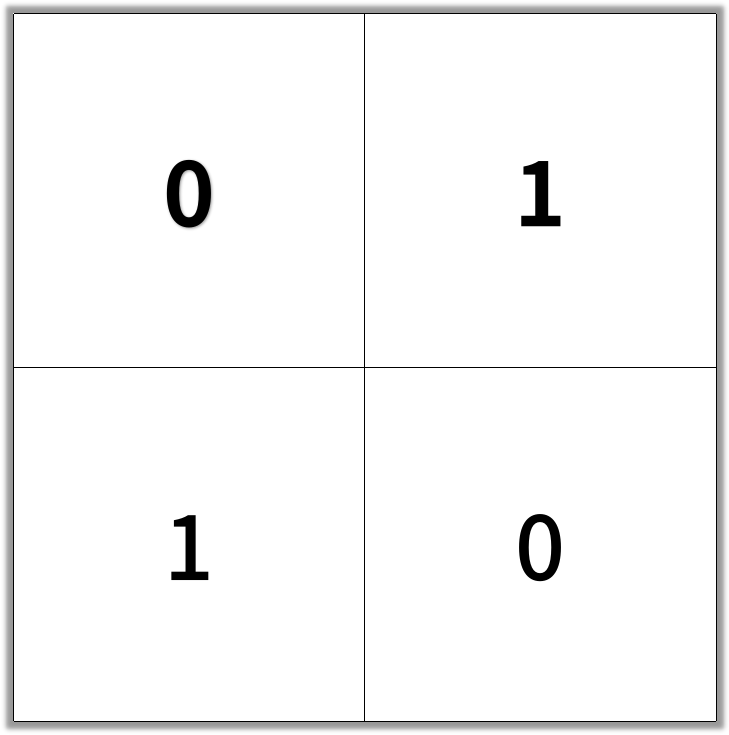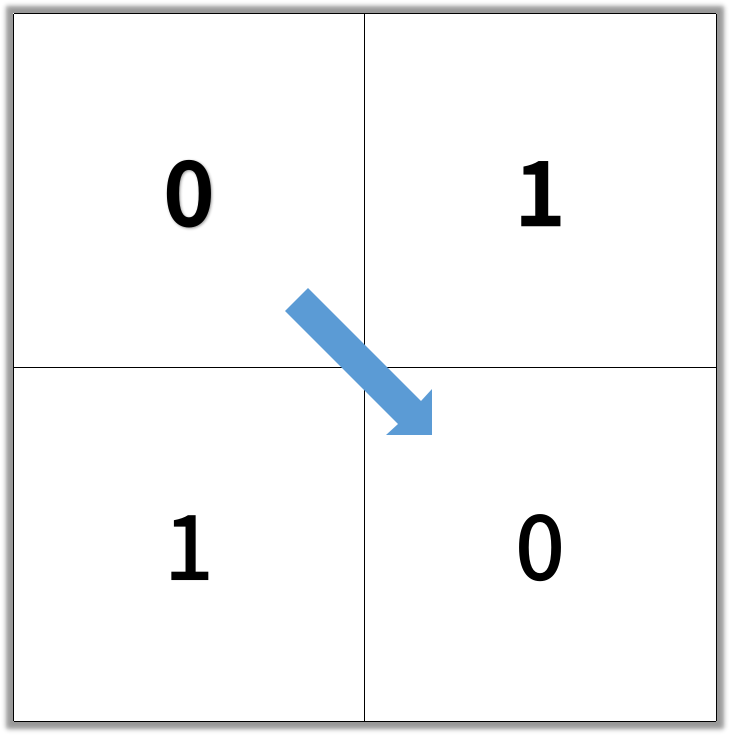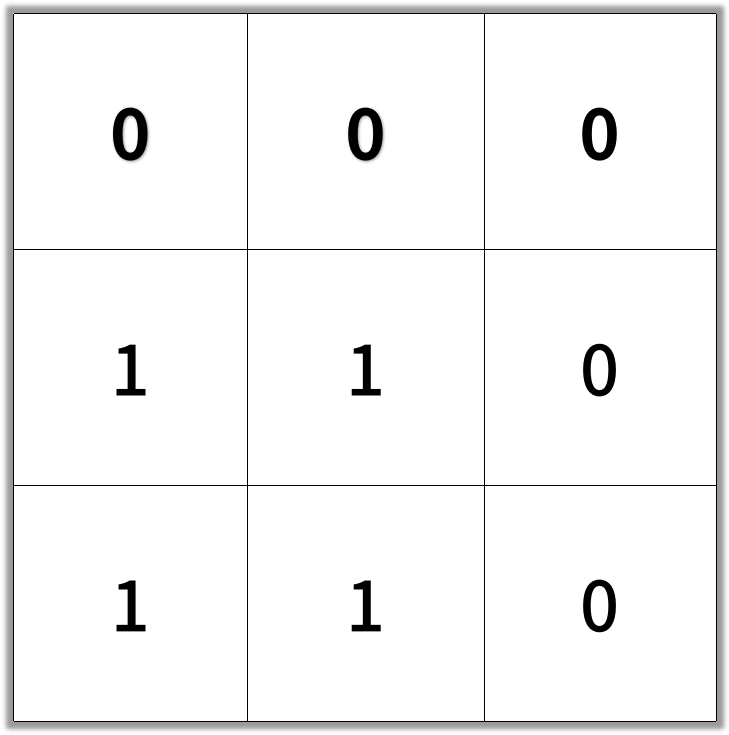##### Welcome to Subscribe On Youtube

Formatted question description: https://leetcode.ca/all/1091.html

# 1091. Shortest Path in Binary Matrix

Medium

## Description

In an N by N square grid, each cell is either empty (0) or blocked (1).

A clear path from top-left to bottom-right has length k if and only if it is composed of cells C_1, C_2, ..., C_k such that:

• Adjacent cells C_i and C_{i+1} are connected 8-directionally (ie., they are different and share an edge or corner)
• C_1 is at location (0, 0) (ie. has value grid)
• C_k is at location (N-1, N-1) (ie. has value grid[N-1][N-1])
• If C_i is located at (r, c), then grid[r][c] is empty (ie. grid[r][c] == 0).

Return the length of the shortest such clear path from top-left to bottom-right. If such a path does not exist, return -1.

Example 1:

Input: [[0,1],[1,0]]Output: 2Example 2:

Input: [[0,0,0],[1,1,0],[1,1,0]]Output: 4Note:

1. 1 <= grid.length == grid.length <= 100
2. grid[r][c] is 0 or 1

## Solution

If grid has 0 rows or 0 columns, then such a path does not exist. Also if the top-left corner of grid is blocked or the bottom-right corner of grid is blocked, then such a path does not exist. In these cases, return -1.

Use breadth first search to find the length of the shortest path. The top-left corner has path length 1. Starting from the top-left corner, search all adjacent cells that are connected 8-directionally. If an adjacent cell is empty and not visited, update the distance of the adjacent cell and add it to the queue for further search.

If the bottom-right corner can be reached, return the length of the shortest path. Otherwise, return -1.

• class Solution {
public int shortestPathBinaryMatrix(int[][] grid) {
if (grid == null || grid.length == 0 || grid.length == 0)
return -1;
int side = grid.length;
if (grid == 1 || grid[side - 1][side - 1] == 1)
return -1;
final int BLOCK = -1;
final int WHITE = 0;
final int GRAY = 1;
final int BLACK = 2;
int[][] colors = new int[side][side];
int[][] distances = new int[side][side];
for (int i = 0; i < side; i++) {
for (int j = 0; j < side; j++) {
if (grid[i][j] == 0) {
colors[i][j] = WHITE;
distances[i][j] = Integer.MAX_VALUE;
} else {
colors[i][j] = BLOCK;
distances[i][j] = -1;
}
}
}
colors = GRAY;
distances = 1;
int[][] directions = { {-1, -1}, {-1, 0}, {-1, 1}, {0, 1}, {1, 1}, {1, 0}, {1, -1}, {0, -1} };
queue.offer(new int[]{0, 0});
while (!queue.isEmpty()) {
int[] cell = queue.poll();
int row = cell, column = cell;
int distance = distances[row][column];
for (int[] direction : directions) {
int newRow = row + direction, newColumn = column + direction;
if (newRow >= 0 && newRow < side && newColumn >= 0 && newColumn < side && colors[newRow][newColumn] == WHITE) {
colors[newRow][newColumn] = GRAY;
distances[newRow][newColumn] = distance + 1;
queue.offer(new int[]{newRow, newColumn});
}
}
colors[row][column] = BLACK;
}
return distances[side - 1][side - 1] == Integer.MAX_VALUE ? -1 : distances[side - 1][side - 1];
}
}

• // OJ: https://leetcode.com/problems/shortest-path-in-binary-matrix/
// Time: O(N^2)
// Space: O(N^2)
class Solution {
public:
int shortestPathBinaryMatrix(vector<vector<int>>& G) {
if (G == 1) return -1;
int N = G.size();
vector<vector<int>> dist(N, vector<int>(N, INT_MAX));
queue<pair<int, int>> q;
q.emplace(0, 0);
dist = 1;
while (q.size()) {
auto [x, y] = q.front();
q.pop();
for (int dx = -1; dx <= 1; ++dx) {
for (int dy = -1; dy <= 1; ++dy) {
if (dx == 0 && dy == 0) continue;
int a = x + dx, b = y + dy;
if (a < 0 || a >= N || b < 0 || b >= N || G[a][b] == 1 || dist[a][b] != INT_MAX) continue;
dist[a][b] = dist[x][y] + 1;
q.emplace(a, b);
}
}
}
return dist[N - 1][N - 1] == INT_MAX ? -1 : dist[N - 1][N - 1];
}
};

• class Solution:
def shortestPathBinaryMatrix(self, grid: List[List[int]]) -> int:
if grid:
return -1
n = len(grid)
q = deque([(0, 0)])
grid = 1
ans = 0
while q:
ans += 1
for _ in range(len(q)):
i, j = q.popleft()
if (i, j) == (n - 1, n - 1):
return ans
for x in range(i - 1, i + 2):
for y in range(j - 1, j + 2):
if 0 <= x < n and 0 <= y < n and grid[x][y] == 0:
q.append((x, y))
grid[x][y] = 1
return -1

############

# 1091. Shortest Path in Binary Matrix
# https://leetcode.com/problems/shortest-path-in-binary-matrix/

class Solution:
def shortestPathBinaryMatrix(self, grid: List[List[int]]) -> int:
rows, cols = len(grid), len(grid)
if grid == 1: return -1

queue = collections.deque([(0, 0, 1)])
visited = set()

while queue:
x, y, steps = queue.popleft()
if x == rows - 1 and y == cols - 1: return steps

for dx in range(x - 1, x + 2):
for dy in range(y - 1, y + 2):
if 0 <= dx < rows and 0 <= dy < cols and grid[dx][dy] == 0 and (dx, dy) not in visited:
queue.append((dx, dy, steps + 1))

return -1


• func shortestPathBinaryMatrix(grid [][]int) int {
if grid == 1 {
return -1
}
n := len(grid)
q := [][]int{[]int{0, 0} }
grid = 1
ans := 0
for len(q) > 0 {
ans++
for m := len(q); m > 0; m-- {
p := q
q = q[1:]
i, j := p, p
if i == n-1 && j == n-1 {
return ans
}
for x := i - 1; x <= i+1; x++ {
for y := j - 1; y <= j+1; y++ {
if x >= 0 && x < n && y >= 0 && y < n && grid[x][y] == 0 {
q = append(q, []int{x, y})
grid[x][y] = 1
}
}
}
}
}
return -1
}

• use std::collections::VecDeque;
impl Solution {
pub fn shortest_path_binary_matrix(mut grid: Vec<Vec<i32>>) -> i32 {
let n = grid.len();
let mut queue = VecDeque::new();
queue.push_back([0, 0]);
let mut res = 0;
while !queue.is_empty() {
res += 1;
for _ in 0..queue.len() {
let [i, j] = queue.pop_front().unwrap();
if grid[i][j] == 1 {
continue;
}
if i == n - 1 && j == n - 1 {
return res;
}
grid[i][j] = 1;
for x in -1..=1 {
for y in -1..=1 {
let x = x + i as i32;
let y = y + j as i32;
if x < 0 || x == n as i32 || y < 0 || y == n as i32 {
continue;
}
queue.push_back([x as usize, y as usize]);
}
}
}
}
-1
}
}


• function shortestPathBinaryMatrix(grid: number[][]): number {
if (grid) {
return -1;
}
const n = grid.length;
grid = 1;
let q: number[][] = [[0, 0]];
for (let ans = 1; q.length > 0; ++ans) {
const nq: number[][] = [];
for (const [i, j] of q) {
if (i === n - 1 && j === n - 1) {
return ans;
}
for (let x = i - 1; x <= i + 1; ++x) {
for (let y = j - 1; y <= j + 1; ++y) {
if (x >= 0 && x < n && y >= 0 && y < n && !grid[x][y]) {
grid[x][y] = 1;
nq.push([x, y]);
}
}
}
}
q = nq;
}
return -1;
}